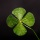Home Communities
IT Knowledge
Inspiration
Languages
EN

# JavaScript - random number between MIN and MAX value - both inclusive

3 pointslena
654

How to generate random number in JavaScript between min and max value?
The condition - both MIN and MAX value have to be inclusive.
[0, 3] - both inclusive (where 0 is min and 3 is max).

1 pointslena
654

Quick solution with usage example:

``````// ONLINE-RUNNER:browser;

// [min, max]
// inclusive min
// inclusive max
function randomNumber(min, max) {
return Math.floor(Math.random() * (max - min + 1) + min);
}

for (var i = 0; i < 15; i++) {
var randomNum = randomNumber(0, 3);
console.log(randomNum);
}``````

Output (note that we use Random so the output each time will be different :D, or there is high probability it will be):

``````3
0
3
3
1
1
1
0
1
3
1
2
1
3
3``````